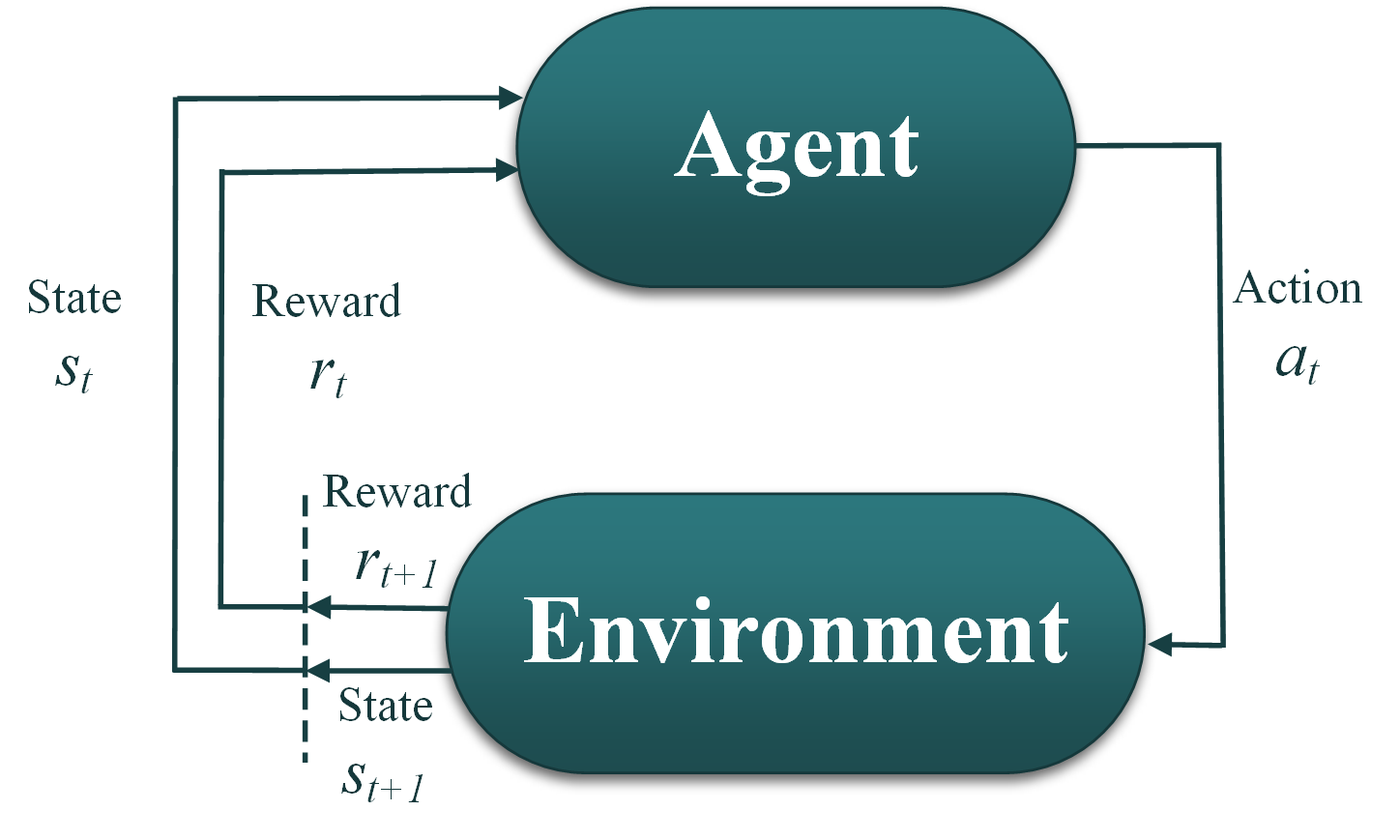# 4. Reinforcement Learning¶

Reinforcement learning (RL) is a paradigm of machine learning concerned with developing intelligent systems, that know how to take actions in an environment in order to maximize cumulative reward. RL does not need labelled input/output data as other machine learning algorithms. Instead, RL collects the data on-the-fly as needed to maximize the reward. This advantage makes RL a natural choice for optimization problems, for which the search space is usually too complex and too high to generate a representative dataset.RL algorithms, like evolutionary algorithms, focus on finding a balance between exploration (new knowledge) and exploitation (of current knowledge) to maximize the fitness/reward function. We can take this analogy to make RL intuitive in solving optimization problems through:

1- The agent: which is the optimizer. The agent is controlled by the RL algorithm that trains the agent to take proper actions. The algorithm takes the current state ($$s_t$$) and the current reward ($$r_t$$) as inputs, and decides the next action to take ($$a_t$$) as output. The action $$a_t$$ in this case is a sample drawn from the parameter space for optimization ($$\vec{x}=[x_1, x_2, ..., x_d]$$).

2- The current state ($$s_t$$) for optimization can be set equal to the current action ($$s_t \leftarrow a_t$$), since we perturb the whole action space at once, and we are not marching through time.

3- The reward is similar as the fitness function in optimization. If it is a minimization problem, the user can convert to reward maximization by multiplying the final fitness value with -1.

4- The environment: takes the action provided by the agent ($$a_t$$), evaluates that action using the fitness function, assigns the next state and the next reward for taking that action ($$s_{t+1}, r_{t+1}$$), and sends them back to the RL agent. In NEORL, the user only needs to specify the fitness function and the parameter space, and NEORL can automatically create the environment class and connect that with the RL agent.

5- Steps 1-4 are repeated for sufficient time steps until the agent learns how to take the right action based on the given state such that the reward is maximized.

6-The best action taken by the agent represents the optimized input ($$\vec{x}$$), while the best reward is similar to the best fitness, $$y=f(\vec{x})$$.

Currently we have a support of some RL algorithms and hybrid neuroevolution, some are listed below

## 4.1. Deep Q Learning¶

See the DQN section

## 4.2. Proximal Policy Optimization¶

See the PPO section